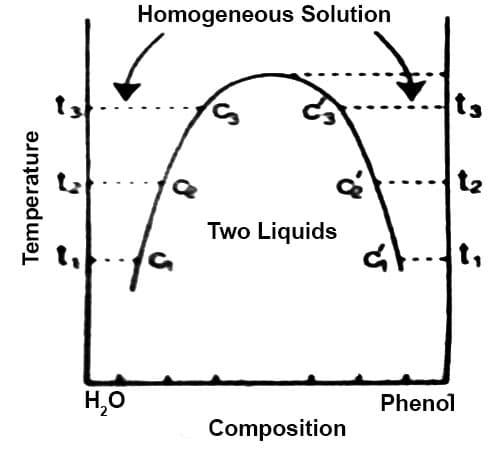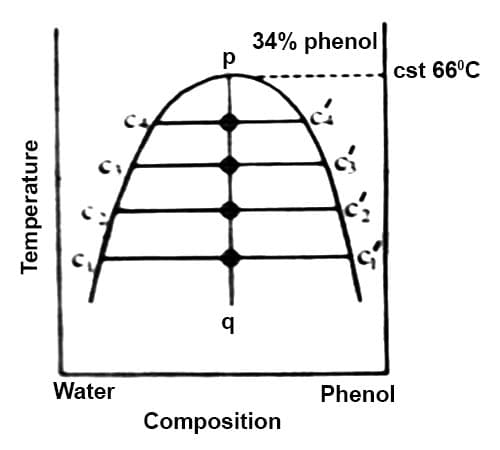Chemistry

# Liquid-Liquid Equilibria in Partially- Miscible SystemsLiquid-Liquid Equilibria in Partially- Miscible Systems: Critical Solution Temperature (CST)

There are pairs of substances which are liquids at ordinary temperatures or whose melting points are low or are known to have limited mutual solubility within certain temperature limits. They are said to be partly miscible in the temperature limits and their solubility curves form an interesting aspect of the application of the phase rule. These substances become completely miscible beyond the temperature limits. These limits are sometimes attained on raising the temperature or sometimes on lowering the temperature. In some systems there are two limits, one higher and the other lower. In this section an attempt will be made to discuss Phenol-water systems.

Phenol-water system: If a small quantity of phenol is added to water at the ordinary temperature the phenol dissolves completely and a clear solution results. On further addition of phenol the dissolution goes on until around 7% of phenol is in solution when a turbid mixture, resembling an emulsion, is obtained. If the mixture is allowed to stand two liquid layers separate out – the upper layer is a solution of phenol in water and the lower layer a solution of water in phenol. If this mixture is slowly heated, at a certain temperature the two liquids again form a clear solution and complete miscibility results. Let this temperature be t1 and the composition c1. Let a little more phenol be added when the computation becomes c2 and the turbidity again appears. The turbid mixture is again heated and at temperature t2 the system again becomes completely miscible. In this way a complete solubility curve can be obtained. If all the points so obtained are plotted a t-c curve results as shown in Figure 1.Figure: t-c diagram of phenol – water system

At each temperature there are two compositions, say c1 and c′2 one representing the composition of phenol in water (c1, c2 etc.) and the other in a solution of water in phenol (c′1, c′2 etc.). These solutions shown by c1 and c′1, c2 and c′2 etc. are called conjugate solutions.

As the temperature is raised to t2, t3 etc. c2 and c′2, c3 and c′3 approach each other until at 660C the two curses meet and complete miscibility results. At 660C and upwards the two components are completely miscible in all proportions. This temperature is called the critical solution temperature or consolute temperature (CST).

The limiting composition of the solution at the critical solution temperature is difficult to evaluate from the graph because the composition axis near the consolute temperature is somewhat flat. The computation is best done by joining the composition of conjugate (figure 2) solutions c1 and c′1, c2 and c′2 etc. by horizontal lines c1, c′1, c2, c′2 etc. The horizontal lines are called tie lines.Fig: t – c diagram of water-phenol